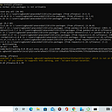# A Python library for basic statistical tests

• One-sample proportion confidence interval
• One-sample proportion significant test
• Two-sample proportion significant test
• One-sample mean confidence interval
• One-sample test of mean
• Two-sample test of mean
• Multi-sample test of mean
• Paired-sample test of mean
• Correlation coefficient and non-correlation test for two continuous variables
• Chi-squred test for two categorical variables
• Mutual information of two categorical variables

# To install

`python setup.py install`
`pip install stat-tests`

# To use

`>>> import stat_tests as st`

## One-sample proportion confidence interval

`>>> st.one_sample_proportion_confidence_interval(n_successes=200, n_trials=1000, confidence=0.95)(0.17520819870781754, 0.22479180129218249)`

## One-sample proportion significant test

`>>> z_score, p_value = st.one_sample_proportion_test(n_successes=5123, n_trials=10000, p_hypo=0.5)>>> z_score, p_value(2.460744684807139, 0.013864899319339763)`
`>>> z_score, p_value = st.one_sample_proportion_test(n_successes=5123, n_trials=10000, p_hypo=0.5, one_side=True)>>> z_score, p_value(2.460744684807139, 0.0069324496596698815)`

## Two-sample proportion significant test

`>>> z_score, p_value = st.two_sample_proportion_test(n_successes_1=20, n_trials_1=300, n_successes_2=21, n_trials_2=298)>>> z_score, p_value(-0.18400991456652802, 0.85400567703455788)`

## One-sample mean confidence interval

`>>> data = 1.80 + 0.2 * np.random.randn(1000)>>> st.one_sample_mean_confidence_interval(data, confidence=0.95)(1.7980145129603369, 1.8228186217572906)`

## One-sample test of mean

`>>> data = 1.80 + 0.2 * np.random.randn(1000)>>> t_score, p_value = st.one_sample_mean_test(data, p_hypo=1.78)>>> t_score, p_value(4.8430777499530517, 1.4808428763412875e-06)`

## Two-sample test of mean

`>>> data1 = 1.80 + 0.2 * np.random.randn(1000)>>> data2 = 1.70 + 0.2 * np.random.randn(1000)>>> t_score, p_value = st.two_sample_mean_test(data1, data2)>>> t_score, p_value(11.720297373853812, 9.9185358995527835e-31)`

## Multi-sample test of mean

`# insignificant case>>> data1 = 1.80 + 0.3 * np.random.randn(1000)>>> data2 = 1.80 + 0.3 * np.random.randn(1000)>>> data3 = 1.80 + 0.3 * np.random.randn(1000)>>> data_sets = (data1, data2, data3)>>> f_score, p_value = st.multi_sample_mean_test(data_sets)>>> f_score, p_value(0.28181526543047031, 0.75443302736823015)# significant case>>> data1 = 1.82 + 0.3 * np.random.randn(1000)>>> data2 = 1.80 + 0.3 * np.random.randn(1000)>>> data3 = 1.85 + 0.3 * np.random.randn(1000)>>> data_sets = (data1, data2, data3)>>> f_score, p_value = st.multi_sample_mean_test(data_sets)>>> f_score, p_value(12.397923609923124, 4.343121211676659e-06)`

## Paired-sample test of mean

`>>> data1 = 1.80 + 0.2 * np.random.randn(1000)# insignificant case>>> data2 = data1 + 0.01 * np.random.randn(len(data1))>>> t_score, p_value = st.paired_sample_mean_test(data1, data2)>>> t_score, p_value(-0.43398665574510392, 0.66439183811383029)# significant case>>> data2 = data1 + 0.01 + 0.01 * np.random.randn(len(data1))>>> t_score, p_value = st.paired_sample_mean_test(data1, data2)>>> t_score, p_value(-34.149842899654423, 5.452067026533736e-170)`

## Correlation coefficient and non-correlation test for two continuous variables

`>>> data1 = 3 + 0.2 * np.random.randn(1000)# insignificant case>>> data2 = 3 + 0.2 * np.random.randn(1000)>>> r, p_value = st.correlation_coef(data1, data2)>>> r, p_value(0.0031232102929028708, 0.92142308109769133)# significant case>>> data2 = data1 + 0.5 * np.random.randn(len(data1))>>> r, p_value = st.correlation_coef(data1, data2)>>> r, p_value(0.37799832675973383, 2.566197851682784e-35)`

## Chi-squred test for two categorical variables

`>>> data1 = np.random.choice(4, 1000)# insignificant case>>> data2 = np.random.choice(2, 1000)>>> st.make_contingency(data1, data2)data1    0    1    2    3data2                    0      126  136  121  1301      115   96  131  145>>> chi2, p_value = st.chisq(data1, data2)>>> chi2, p_value(2.3626413559788064, 0.50062680584524433)# significant case>>> data2 = [np.random.choice(2, p=(0.9, 0.1)) if i in (0, 2) else np.random.choice(2, p=(0.1, 0.9)) for i in data1]>>> st.make_contingency(data1, data2)data1    0    1    2    3data2                    0      209   27  226   251       32  205   26  250>>> chi2, p_value = st.chisq(data1, data2)>>> chi2, p_value(609.12165019624854, 1.0614940711031585e-131)`

## Mutual information of two categorical variables

`>>> data1 = np.random.choice(4, 1000)# insignificant case>>> data2 = np.random.choice(2, 1000)>>> mutual_information = st.mutual_information(data1, data2)>>> mutual_information0.0017608733794212128# significant case>>> data2 = [np.random.choice(2, p=(0.9, 0.1)) if i in (0, 2) else np.random.choice(2, p=(0.1, 0.9)) for i in data1]>>> mutual_information = st.mutual_information(data1, data2)>>> mutual_information0.32882984773406321`

--

--

--

## More from Yang Zhang

Software Engineering SMTS at Salesforce Commerce Cloud Einstein

Love podcasts or audiobooks? Learn on the go with our new app.

## Big data & small files problem in HDFS## What promotion to send to your customer? Hear what data has to say.## Analysing survey data with Python and Jupyter Notebooks## Chronic failure stories for better component reliability using Python## The Battle of Neighborhoods | Finding a Better Place in Scarborough, Toronto## How Floodlight Works## Machine Learning for Financial Analysis## Data Mining## Yang Zhang

Software Engineering SMTS at Salesforce Commerce Cloud Einstein

## Cracking regression analysis: basic steps using Python.## How to Perform One-Hot Encoding the Right Way Using Pandas## PULLING FINANCIAL DATASET USING YAHOO FINANCE API IN PYTHON## Analyse Heart Attacks with Descriptive Parameters